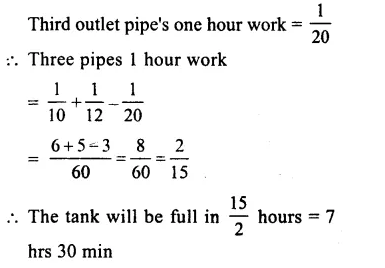# RS Aggarwal Class 8 Solutions Chapter 13 Time and Work Ex 13B

## RS Aggarwal Class 8 Solutions Chapter 13 Time and Work Ex 13B

These Solutions are part of RS Aggarwal Solutions Class 8. Here we have given RS Aggarwal Solutions Class 8 Chapter 13 Time and Work Ex 13B.

Other Exercises

Question 1.
Solution:
A’s 1 day’s work = $$\\ \frac { 1 }{ 10 }$$
B’s 1 day’s work = $$\\ \frac { 1 }{ 15 }$$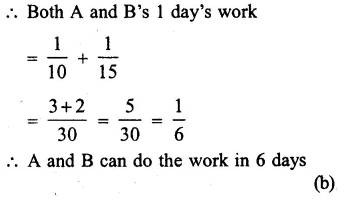Question 2.
Solution:
A man’s 1 day work = $$\\ \frac { 1 }{ 5 }$$
Man and his son’s 1 days work = $$\\ \frac { 1 }{ 3 }$$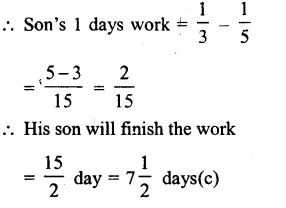Question 3.
Solution:
A’s 1 day’s work = $$\\ \frac { 1 }{ 16 }$$
B’s 1 day’s work = $$\\ \frac { 1 }{ 12 }$$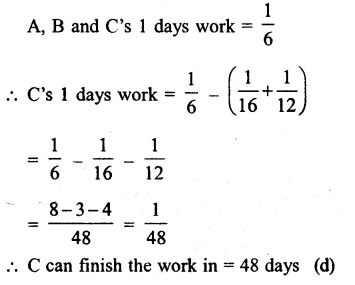Question 4.
Solution:
Let B can do a work in = x days
Then A can do the work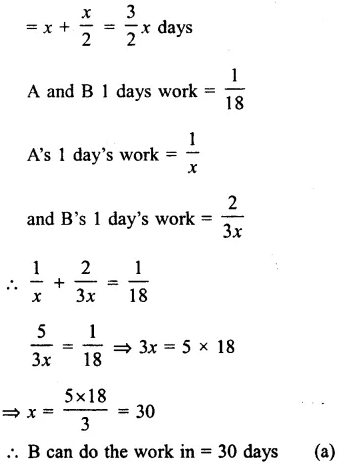Question 5.
Solution:
Let B’s 1 day’s work = x
Then A’s 1 day’s work = 2x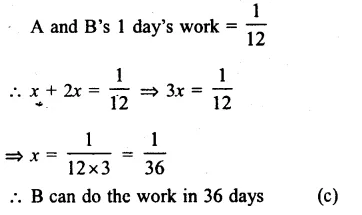Question 6.
Solution:
Total wages = Rs. 3000
A’s 1 day’s work = $$\\ \frac { 1 }{ 10 }$$
B’s 1 day’s work = $$\\ \frac { 1 }{ 15 }$$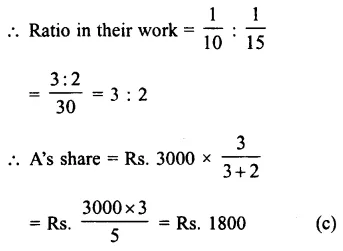Question 7.
Solution:
Ratio in the rates of working of A and B = 3:4
Ratio in time = $$\\ \frac { 1 }{ 3 }$$ : $$\\ \frac { 1 }{ 4 }$$
= $$\\ \frac { 4:3 }{ 12 }$$
= 4 : 3 (c)

Question 8.
Solution:
A and B’s 1 day’s wok = $$\\ \frac { 1 }{ 12 }$$
B and C’s 1 day’s work = $$\\ \frac { 1 }{ 20 }$$
C and A’s 1 day’s work = $$\\ \frac { 1 }{ 15 }$$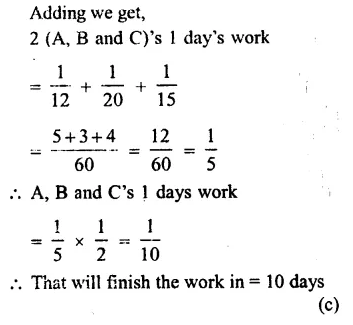Question 9.
Solution:
3 men = 5 women
1 man = $$\\ \frac { 5 }{ 3 }$$ women 5
6 men = $$\\ \frac { 5 }{ 3 }$$ x 6 = 10 women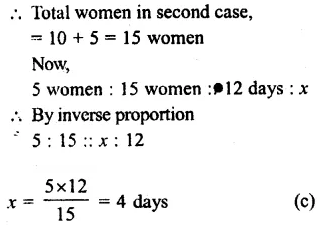Question 10.
Solution:
A’s 1 day’s work = $$\\ \frac { 1 }{ 15 }$$
Then B’s 1 day’s work = $$\\ \frac { 1 }{ 10 }$$ x $$\\ \frac { 100+50 }{ 100 }$$
= $$\\ \frac { 1 }{ 15 }$$ x $$\\ \frac { 150 }{ 100 }$$
= $$\\ \frac { 1 }{ 10 }$$
B will finish the work in = 10 days (a)

Question 11.
Solution:
A’s 1 hour’s work = $$\\ \frac { 2 }{ 15 }$$
A and B’s ratio in work = $$\\ \frac { 100-20 }{ 100 }$$ : 1
= $$\\ \frac { 80 }{ 100 }$$ : 1
= $$\\ \frac { 4 }{ 5 }$$ : 1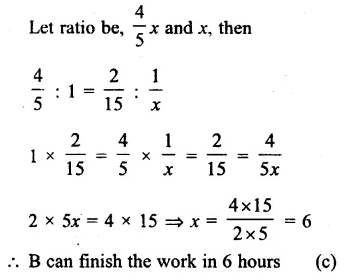Question 12.
Solution:
A’s 1 day’s work = $$\\ \frac { 1 }{ 20 }$$
B’s 1 day’s work = $$\\ \frac { 1 }{ 12 }$$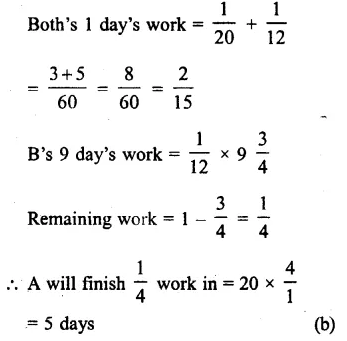Question 13.
Solution:
A’s 1 days work = $$\\ \frac { 1 }{ 25 }$$
B’s 1 days work = $$\\ \frac { 1 }{ 20 }$$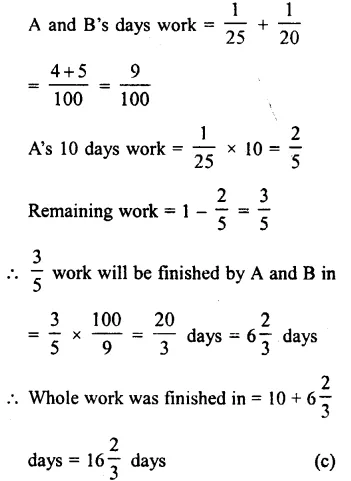Question 14.
Solution:
First pipe 1 minutes work = $$\\ \frac { 1 }{ 20 }$$
Second pipe 1 minutes work = $$\\ \frac { 1 }{ 30 }$$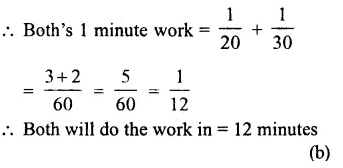Question 15.
Solution:
First tap’s 1 hours work to fill = $$\\ \frac { 1 }{ 8 }$$
Second tap’s 1 hours work to empty = $$\\ \frac { 1 }{ 16 }$$
Both 1 hour can fill the cistern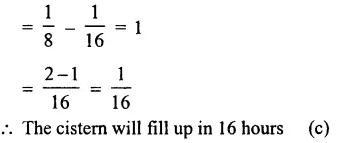Question 16.
Solution:
First pump’s 1 hr work to fill = $$\\ \frac { 1 }{ 2 }$$
Due to leakage, tank is filled in $$2\frac { 1 }{ 3 }$$ hour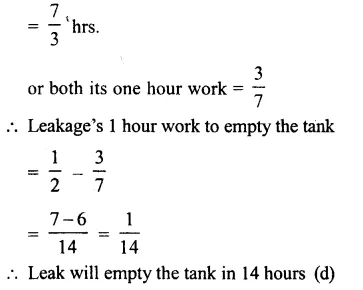Question 17.
Solution:
First inlet pipe’s 1 hour work = $$\\ \frac { 1 }{ 10 }$$
Second inlet pipe’s 1 hour work = $$\\ \frac { 1 }{ 12 }$$